##### The Japanese Bonsai specialist
Direct order Contact Help / Services Newsletter# Fertilizer for bonsai and acer basacote high k 6m

› Bonsai soil and fertiliser › Fertilizer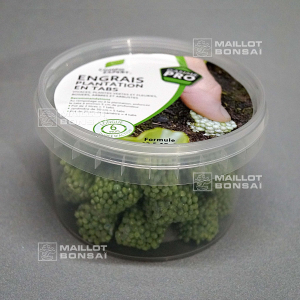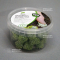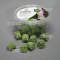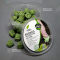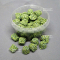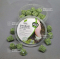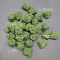ref. : 10502

11,30

Available quantity : 16Order

###### Description

Basacote© High K 6M 13.5.18 plantation fertilizer.

Slow difusion pellets, active for 6 months. Trees and shrubs, bonsaï, perrenials, foliage and flower plants. The plantation fertilizer, based of Basacote©, is a complete fertilizer (Boron, copper, iron, manganese, molybdenum, zinc). Fertilizer poor in chlorine with a coating that guarantees a 6 months activity. Fertilizer for professionals, Basacote© is used by nursery men and incorporated in substrates by compost makers. Basacote© plantation fertilizer can be used : in spring or in fall during the plantation of ornamentation trees, fruit trees, grapevine, shrubs, perrenials plants and bonsaï. It can also be used as maintenance manure : at the base of the trees and the shrubs, all along the year and after plantation. How to use the product for plantation : put on the ground or bury a tabs for 2 litres of soil or 5 tabs for a pot of 50 cm and then cover it with a little of soil (+/- 1 cm). Do not put the product in direct contact with naked roots. In case of renewing the surface layer, after application hallow the soil to bury briefly the pellets. Before repoting : mix 2 to 4 pellets in a bucket for 4 to 5 litres of compost. A bucket of Basacote© can fertilize 80 litres of soil. A Basacote© bucket contains about 40 tabs or 300 grams. A regular use of Basacote© will help you to avoid a lot of maintenance and cultivation problems by helping a vigorous plant to better resist to many exterior attacks.

#fertilizer 11 #basacote 9.8 #plantation 7 #bonsai 4.6 #trees 4.5 #soil 4.4 #pellets 3.7 #shrubs 3.6 #litres 3.6 #bucket 3.6

Formule
(( ROUND((CHAR_LENGTH(b.article_nom)-CHAR_LENGTH(REPLACE(b.article_nom, 'basacote', '')))/LENGTH('basacote')) + ROUND((CHAR_LENGTH(b.article_description)-CHAR_LENGTH(REPLACE(b.article_description, 'basacote', '')))/LENGTH('basacote')) ) * 9.8) + (( ROUND((CHAR_LENGTH(b.article_nom)-CHAR_LENGTH(REPLACE(b.article_nom, 'fertilizer', '')))/LENGTH('fertilizer')) + ROUND((CHAR_LENGTH(b.article_description)-CHAR_LENGTH(REPLACE(b.article_description, 'fertilizer', '')))/LENGTH('fertilizer')) ) * 9) + (( ROUND((CHAR_LENGTH(b.article_nom)-CHAR_LENGTH(REPLACE(b.article_nom, 'plantation', '')))/LENGTH('plantation')) + ROUND((CHAR_LENGTH(b.article_description)-CHAR_LENGTH(REPLACE(b.article_description, 'plantation', '')))/LENGTH('plantation')) ) * 7) + (( ROUND((CHAR_LENGTH(b.article_nom)-CHAR_LENGTH(REPLACE(b.article_nom, 'bonsai', '')))/LENGTH('bonsai')) + ROUND((CHAR_LENGTH(b.article_description)-CHAR_LENGTH(REPLACE(b.article_description, 'bonsai', '')))/LENGTH('bonsai')) ) * 4.6) + (( ROUND((CHAR_LENGTH(b.article_nom)-CHAR_LENGTH(REPLACE(b.article_nom, 'trees', '')))/LENGTH('trees')) + ROUND((CHAR_LENGTH(b.article_description)-CHAR_LENGTH(REPLACE(b.article_description, 'trees', '')))/LENGTH('trees')) ) * 4.5) + (( ROUND((CHAR_LENGTH(b.article_nom)-CHAR_LENGTH(REPLACE(b.article_nom, 'soil', '')))/LENGTH('soil')) + ROUND((CHAR_LENGTH(b.article_description)-CHAR_LENGTH(REPLACE(b.article_description, 'soil', '')))/LENGTH('soil')) ) * 4.4) + (( ROUND((CHAR_LENGTH(b.article_nom)-CHAR_LENGTH(REPLACE(b.article_nom, 'pellets', '')))/LENGTH('pellets')) + ROUND((CHAR_LENGTH(b.article_description)-CHAR_LENGTH(REPLACE(b.article_description, 'pellets', '')))/LENGTH('pellets')) ) * 3.7) + (( ROUND((CHAR_LENGTH(b.article_nom)-CHAR_LENGTH(REPLACE(b.article_nom, 'shrubs', '')))/LENGTH('shrubs')) + ROUND((CHAR_LENGTH(b.article_description)-CHAR_LENGTH(REPLACE(b.article_description, 'shrubs', '')))/LENGTH('shrubs')) ) * 3.6) + (( ROUND((CHAR_LENGTH(b.article_nom)-CHAR_LENGTH(REPLACE(b.article_nom, 'litres', '')))/LENGTH('litres')) + ROUND((CHAR_LENGTH(b.article_description)-CHAR_LENGTH(REPLACE(b.article_description, 'litres', '')))/LENGTH('litres')) ) * 3.6) + (( ROUND((CHAR_LENGTH(b.article_nom)-CHAR_LENGTH(REPLACE(b.article_nom, 'bucket', '')))/LENGTH('bucket')) + ROUND((CHAR_LENGTH(b.article_description)-CHAR_LENGTH(REPLACE(b.article_description, 'bucket', '')))/LENGTH('bucket')) ) * 3.6)

## Secure payment## Delivery

Our logistic partners :04 74 55 23 48
Pépinière MAILLOT-BONSAÏ
Le Bois Frazy
01990 RELEVANT - FRANCE
on appointment